# 十步搞定单因素方差分析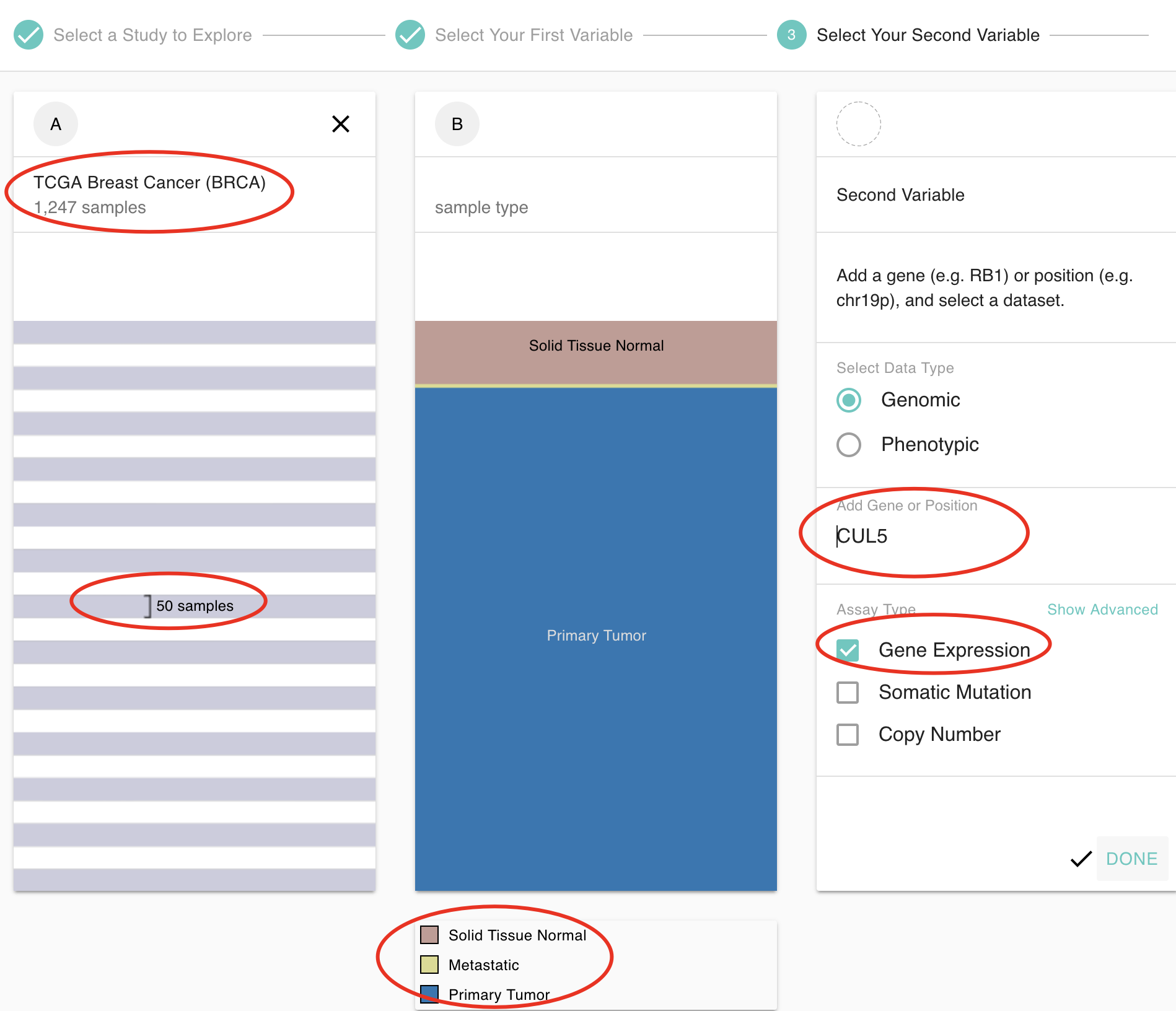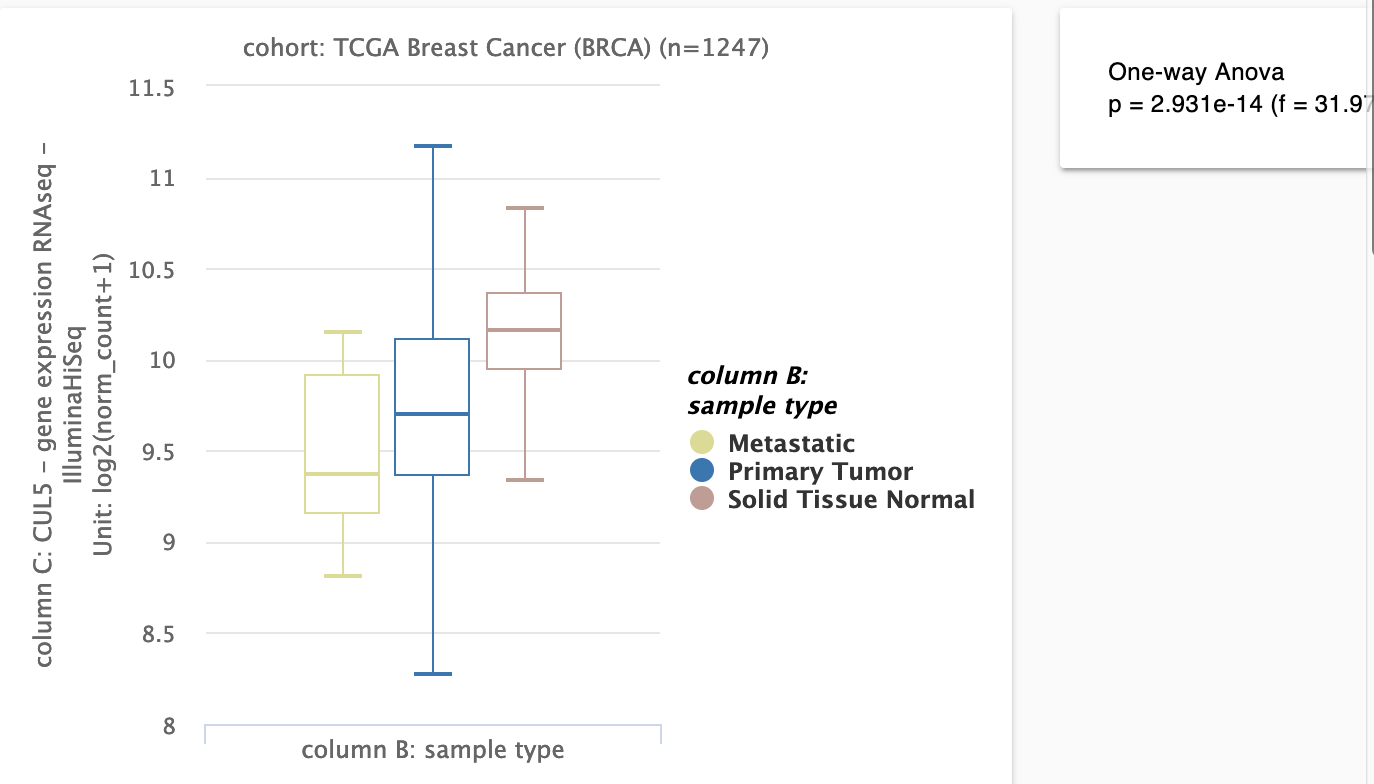### 定义

• 因素：影响研究对象的某一指标变量
• 水平：因素变化的各种状态或因素变化所分的等级或组别。
• 单因素试验：考虑的因素只有一个的试验叫单因素试验。

### 了解数据

数据文件可以下载，然后读入R里面进行可视化，代码如下：

``````rm(list = ls())
options(stringsAsFactors = F)
library(ggpubr)
colnames(df)
p = ggboxplot(df, "sample_type", "CUL5",
color = "sample_type", palette =c("#00AFBB", "#E7B800", "#FC4E07"),
add = "jitter", shape = "sample_type")
p
``````

这个文件 `CUL5-BRCA-type.tsv` 如果你没有看我的TCGA肿瘤数据库知识图谱可能不知道如何下载，可以发邮件给我找我申请这个测试数据 ( 邮箱： jmzeng1314@163.com )
可以看到，比网页工具出图要好看：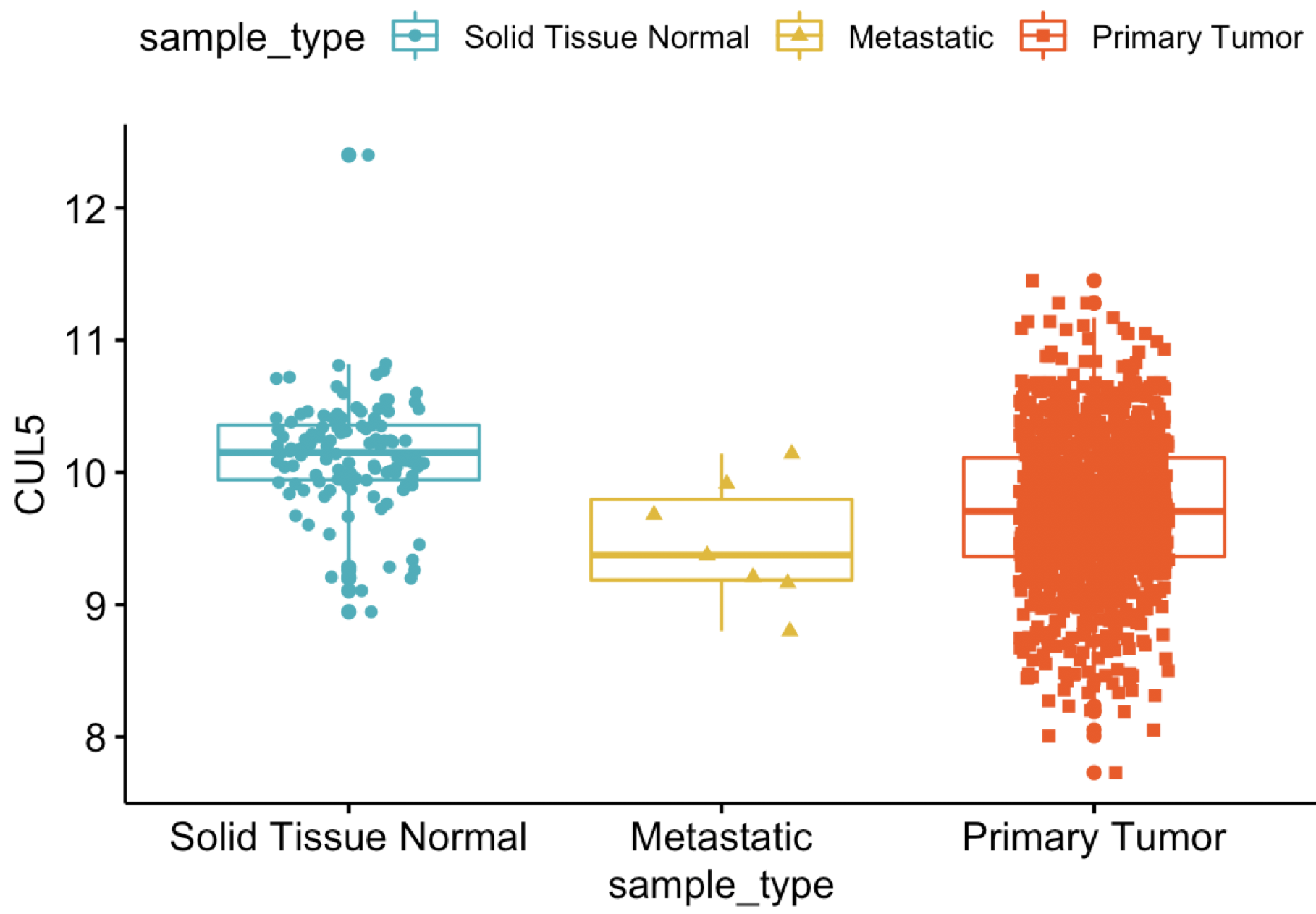但是，这个时候还没有进行统计分析，可以添加的统计学检验包括：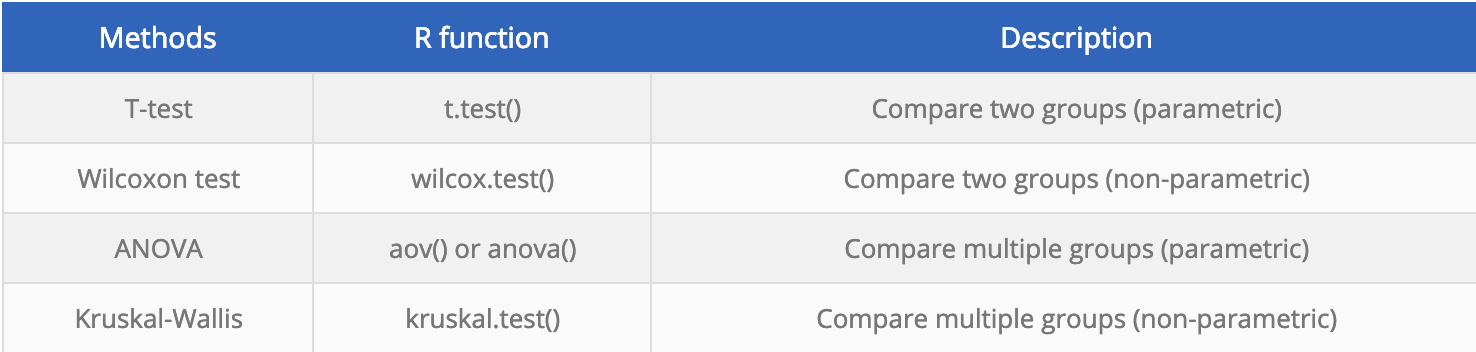代码也很简单：

``````p+stat_compare_means(method = "anova", label.y = 10)+ # Add global p-value
stat_compare_means(label = "p.signif", method = "t.test",
ref.group = ".all.") # Pairwise comparison against all
``````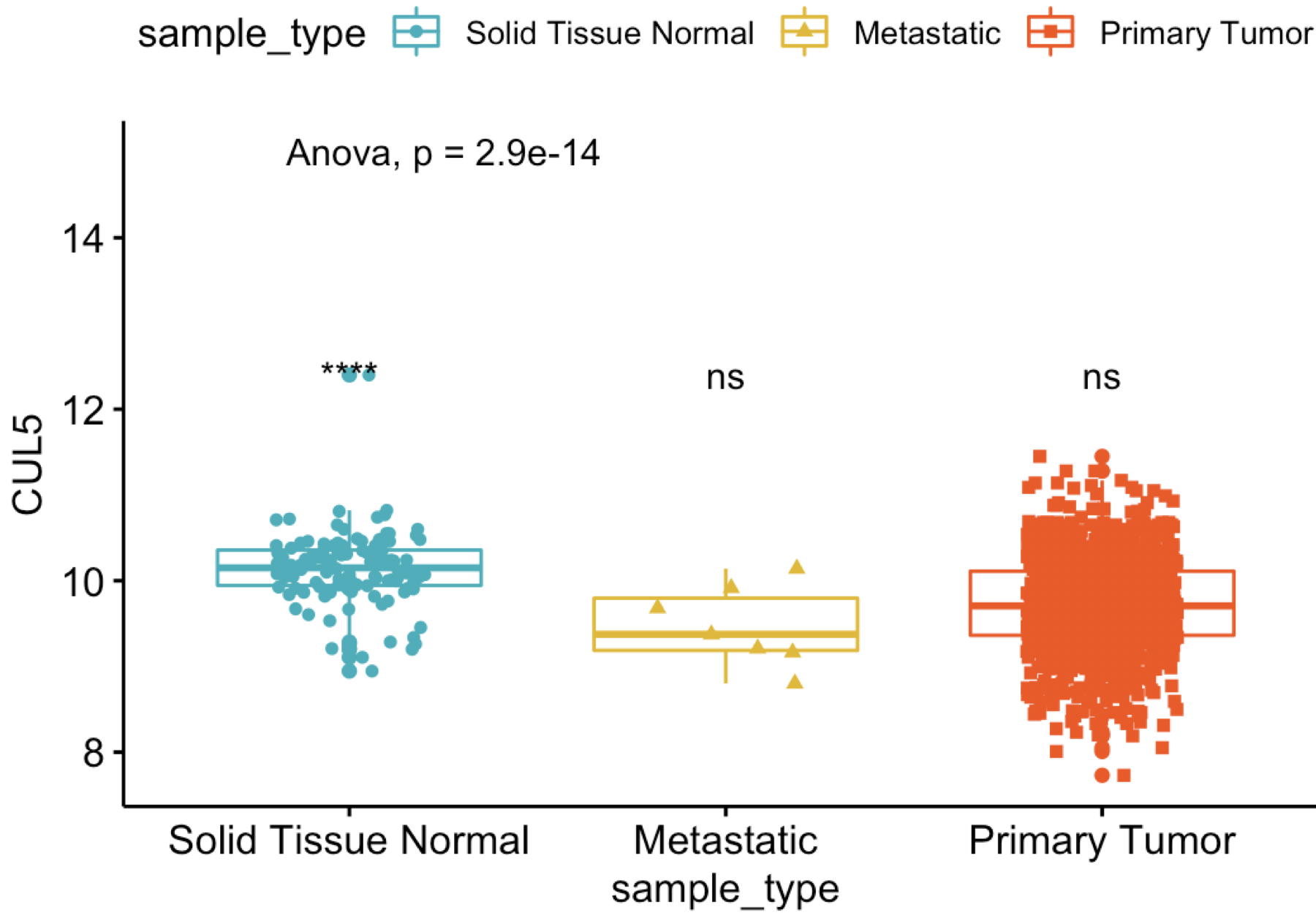可以看到，跟网页工具结果一模一样，而且出图更漂亮，下面我们就手把手带领大家完成这个分析，把这个ggpubr一步就完成的工作拆解开来。

### 第1步：计算各组内样本均值

``````lapply(split(df,df\$sample_type),function(x) mean(x\$CUL5))
``````

### 第2步：计算所有样本均值

``````mean(df\$CUL5 )
``````

### 第3步：计算各组内部误差平方和

``````tmp=lapply(split(df,df\$sample_type),function(x) sum((x\$CUL5-mean(x\$CUL5))^2) )
sse = sum(unlist(tmp))
``````

### 第4步：计算各组间误差平方和

``````tmp=lapply(split(df,df\$sample_type),function(x) nrow(x)*(mean(x\$CUL5) - mean(df\$CUL5 ))^2 )
ssb = sum(unlist(tmp))
``````

### 第5步：计算各组内部均方误

``````mse=sse/(nrow(df)-length(unique(df\$sample_type)))
``````

### 第6步：计算组间均方误

``````msd=ssb/length(unique(df\$sample_type))-1
``````

### 第7步：计算F比率

``````f= msb/mse
f
``````

### 第8步：查找F临界值

``````df1=(length(unique(df\$sample_type))-1)
df2=(nrow(df)-length(unique(df\$sample_type)))
qf(0.05,2,1215)
``````

差别可知这里的F值是0.05，远小于我们真实情况，所以非常显著了。

### 第9步：判断是否显著

``````1-pf(f,2,1215)
``````

现在我们已经知道了，在选定的显著水平为0.05时候，这个F统计是显著的，但是仍然是不知道哪组之间不一样， 所以可以选择tukey检验

### 第10步：进行tukey检验,多重比较

J·W·图凯（Tukey）于1953年提出一种能将所有各对平均值同时比较的方法，这种方法现在已被广泛采用，一般称之为“HSD检验法”，或称“W法”。 Tukey (John Wilder Tukey) for multiple comparisons
主要应用于3组或以上的多重比较。比如说一共有4组数据，两两比较产生6个统计值，Tukey test用于生成一个critical value来控制总体误差（Familywise error rate，FER）;与Tukey test相类似的是Dunnett test，它是控制多对一比较（即3组同时和一个参照组比较）的FER。
这个多重比较算法还蛮多的，参考：https://zhuanlan.zhihu.com/p/44880434